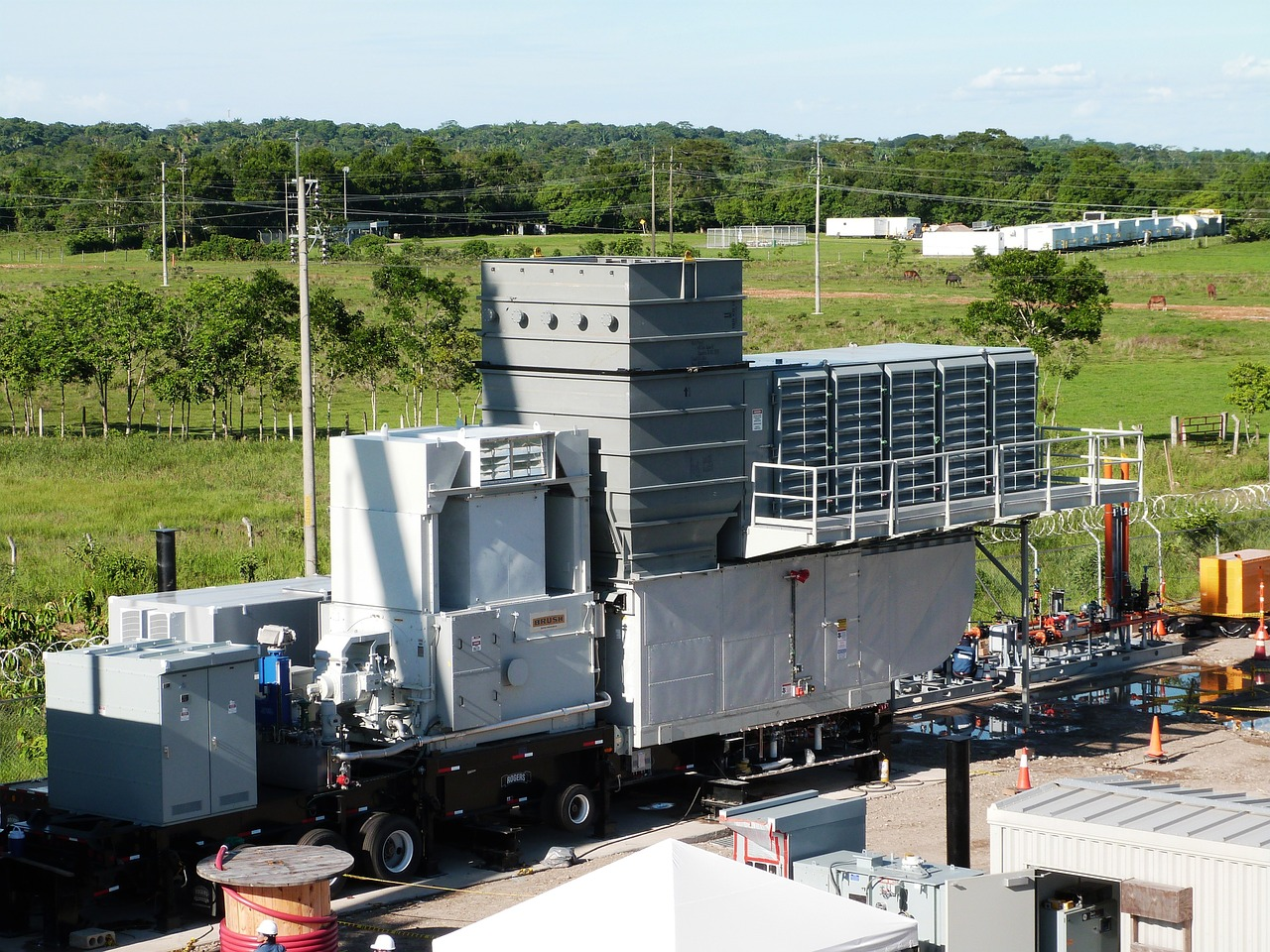# Estimation of Gas Turbine Fuel Rates

In this post I want to share how to estimate gas turbine fuel rates. We use heat rates to estimate gas turbine fuel rates.

Heat rate is a measure of thermal efficiency or the amount of energy (in the form of fuel) which must be input to the gas turbine to produce the output power. Heat rate is usually expressed in terms of Btu/(hp.hr) or Btu/(kW.hr) based on the lower heating value of the fuel. Heat rate and thermal efficiency are related as follows:

Gas turbine heat rates vary considerably. They are function of gas turbine rating and type of turbines, which are simple cycle, jet turbine simple cycle, or regenerative.

We can use graph below for initial estimation of gas turbine heat rates.

Based on graph above, we can see that the higher gas turbine rating, the lower heat rate will be. For the same gas turbine ratings, simple cycle types provide the highest heat rates, on the other hand, regenerative gas turbine require the lowest heat rates.

Power and heat rate both vary depending on environmental conditions such as ambient air temperature, altitude, barometric pressure, and humidity. Therefore, when performance is stated for a gas turbine, the ambient conditions must be stated. To compare different gas turbines, a set of standard conditions known as ISO (International Standards Organization) conditions have been defined as follows:

ISO Conditions:

• Ambient temperature = 59oF = 15oC
• Altitude = 0 ft (sea level)
• Ambient pressure = 29.92 in. Hg (14.7 psi)
• Relative humidity = 0%

All gas turbine performance is stated in ISO conditions. To arrive at site rated horsepowers, the ISO conditions must be corrected for the following:

• Altitude
• Inlet losses : pressure drop which occurs as the outside air passes through the inlet filters and plenum
• Exhaust losses : pressure drop through the exhaust stack, silencers, and heat recovery equipment (if any) which creates a back pressure on the turbine
• Temperature
• Humidity

For humidity, a good rule of thumb is to increase the heat rate by the wt% moisture in the inlet air.

For example, if the inlet air contains 1% moisture, the heat rate correction factor is (1+1% = 1.01). If the inlet air contains 2% moisture, the heat rate correction factor is (1+2% = 1.02), etc. No power correction is normally required.

Performance is also affected by other installation variables including power take-offs and type of fuel used. Power take-offs include any devices such as oil pumps, generators, etc which are directly driven from the gas turbine output shaft and thus reduce the available output power. Sometimes it is necessary to correct power and heat rate for the type of fuel used in the gas turbine. Regarding this topic, we should consult turbine manufacturers.

The following example shows the method of calculating performance for a gas turbine at site conditions using data typically supplied in the manufacturer’s performance brochure.

## Example

A gas turbine has the following specification:

Turbine ISO horsepower               = 27,500

Turbine ISO heat rate                    = 7,090 Btu/(hp-hr)

Ambient temperature                   = 80oF

Altitude                                         = 1000 ft (above sea level)

Inlet pressure drop                       = 4 in H2O

Exhaust pressure drop                  = 2 in H2O

Relative humidity                          = 0%

Fuel                                               = natural gas

What is maximum available site power and heat rates?

## Solution

We need to find correction factors for power and heat rate.

## Correction factors for power

As mentioned above, correction factors include correction factor for altitude, exhaust loss, inlet loss, ambient temperature, relative humidity, and type of fuel. Let’s check the correction factor values for power. From graphs we find:

• Power altitude correction factor (for 1000 ft altitude) = 0.965
• Power exhaust loss correction factor (for 2 in H2O) = 0.9965
• Power inlet loss correction factor (for 4 in H2O) = 0.984
• Power ambient temperature correction factor (for 80oF) = 0.915
• Power relative humidity correction factor (for 0% relative humidity) = no correction factor required
• Power type of fuel correction factor (for natural gas) = no correction factor required

We get maximum available site power by multiplying maximum-no-loss power by each correction factors.

Power (site) = power (0.965) (0.9965) (0.984) (0.915)

Power (site) = 27,500 (0.965) (0.9965) (0.984) (0.915)

Power (site) = 23,800 hp

We do the same for heat rates.

• Heat rate altitude correction factor (for 1000 ft altitude) = no correction factor because heat rate is not affected by altitude
• Heat rate exhaust loss correction factor (for 2 in H2O) = 1.003
• Heat rate inlet loss correction factor (for 4 in H2O) = 1.0065
• Heat rate ambient temperature correction factor (for 80oF) = 1.03
• Heat rate humidity correction factor (for 0% relative humidity) = no correction factor required
• Heat rate type of fuel correction factor (for natural gas) = no correction factor required

We get site heat rate by multiplying no-loss heat rate by the correction factors.

Heat rate (site) = heat rate (1.003) (1.0065) (1.03)

Heat rate (site) = 7090 (1.003) (1.0065) (1.03)

Heat rate (site) = 7370 Btu/(hp-hr)

The above calculation procedure may slightly different with manufacturers, but will follow the same principle.

I hope you find this post useful 😊

References:

1. Branan, Carl, “Rules of Thumb for Chemical Engineers”, Gulf Professional Publishing, 2005
2. GPSA Engineering Data Book